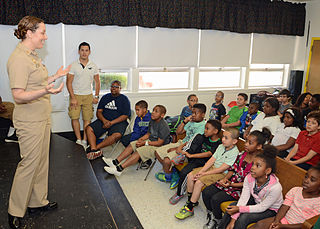# Logical Maths is not the only mathsDuring a meeting from their school mathematics club, Andy, Becky, Chloe and Danny discover that their favourite number (a positive integer) is the same. So, each of the four friends makes three statements about the number, at least one of which is true and at least one of which is false.

$\begin{matrix} \textbf{Andy}: & \textit{(A1)} & \text{The number is less than 12}\\ & \textit{(A2)} & \text{7 does not divide the number}\\ & \textit{(A3)} & \text{5 times the number is less than 70}\\ \textbf{Becky}: & \textit{(B1)} & \text{12 times the number is greater than 1000}\\ & \textit{(B2)} & \text{10 divides the number}\\ & \textit{(B3)} & \text{The number is greater than 100}\\ \textbf{Chloe} : & \textit{(C1)} & \text{4 divides the number}\\ & \textit{(C2)} & \text{11 times the number is less than 1000}\\ & \textit{(C3)} & \text{9 divides the number}\\ \textbf{Danny} : & \textit{(D1)} & \text{The number is less than 20}\\ & \textit{(D2)} & \text{The number is a prime number}\\ & \textit{(D3)} & \text{7 divides the number} \end{matrix}$

What is their favorite number?

×